# Solution assignment 10 Exponential functions and graphs

### Assignment 10

For which value(s) of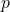do the graphs of the functions: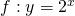have only one point in common.

### Solution

In order to calculate the intersection points ofand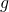we have to solve the following equation: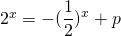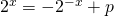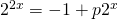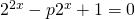Substituting:in the equation we get: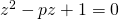This quadratic equation has two coinciding solutions if the discriminant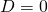, so if: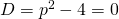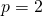or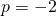Ifwe get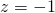, i.e.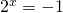and this does not give a solution. Thus the value offor which both graphs have just one common point is.

Verify that the common point is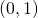, see the figure.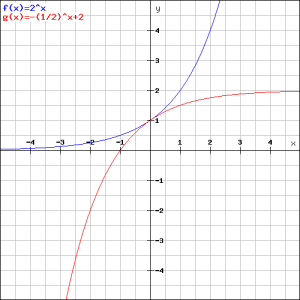0